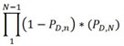## Probability of interruption

The probability of interruption (PI) is the probability that the security system successfully detects the adversary attacking along the given pathway so that the response force is able to successfully engage the adversary and preventing completion of the intended task.

The probability of interruption is calculated by determining the combined detection probability before the CDP. This is found usingWhere:

PD,N = the probability of detection on the Nth task= the probability that the adversary is not detected until the Nth task